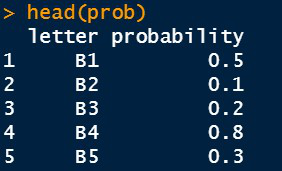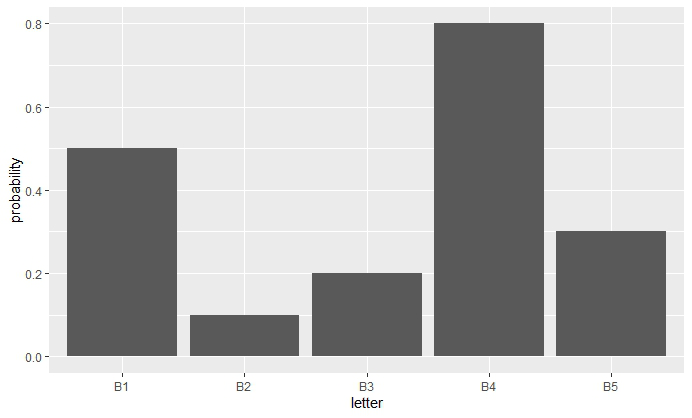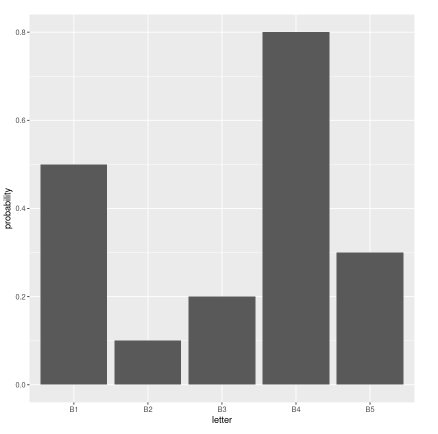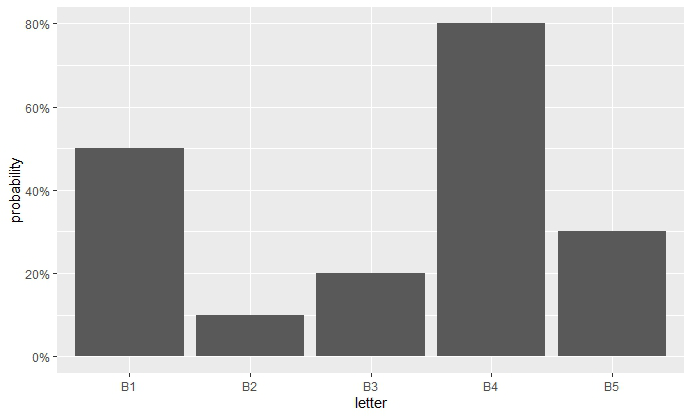# Change Y-Axis to Percentage Points in ggplot2 Barplot in R

• Last Updated : 24 Jun, 2021

In this article, we will discuss how to change the Y-axis to percentage using the ggplot2 bar plot in R Programming Language.

First, you need to install the ggplot2 package if it is not previously installed in R Studio. To install and load write the below command in R Console :

```install.packages("ggplot2")
library(ggplo2)```

For creating a simple bar plot we will use the function geom_bar( ).

Syntax:

geom_bar(stat, fill, color, width)

Parameters :

• stat : Set the stat parameter to identify the mode.
• fill : Represents color inside the bars.
• color : Represents color of outlines of the bars.
• width : Represents width of the bars.

First, we will create a Data Frame which has two vectors “letter” and “probability” and stores it in a variable prob.

## R

 `# Insert Data``prob <- ``data.frame``(letter = ``c``(``"B1"``,``"B2"``,``"B3"``,``"B4"``,``"B5"``),``                   ``probability = ``c``(0.5, 0.1, 0.2, 0.8, 0.3))`` ` `head``(prob)`Let’s create a simple bar plot.

## R

 `# Insert Plot``library``(``"ggplot2"``)`` ` `dt <- ``ggplot``(data=prob, ``aes``(x=letter, y=probability)) +``  ``geom_bar``(stat = ``"identity"``)`` ` `dt`## Changing Y-axis to Percentage

Some important keywords used are :

1. accuracy: The precision value to which a number is round to.
2. scale: It is used for scaling the data. A scaling factor is multiplied with the original data value.
3. labels: It is used to assign labels.

The function used is scale_y_continuous( ) which is a default scale in “y-aesthetics” in the library ggplot2. Since we need to add percentages in the labels of the Y-axis, the keyword “labels” is used.

Now use scales: : percent to convert the y-axis labels into a percentage. This will scale the y-axis data from decimal to percentage. It simply multiplies the value by 100. The scaling factor is 100.

In the above code add :

## R

 `# Changing Y-axis to percentage``dt + ``scale_y_continuous``(labels = scales::percent)`

Output:In older versions of R, using the above code you may get the percentage values having one digit after the decimal in the Y-axis as shown in the above example. In such a case, we will use the function percent_format( ) to modify the accuracy of the percentage labels in Y-axis. It is basically used to assign the precision value in order to round it.

Now, modify the above code into :

## R

 `# Accuracy of y-axis``dt + ``scale_y_continuous``(labels = scales::``percent_format``(accuracy = 1))`

Output:My Personal Notes arrow_drop_up